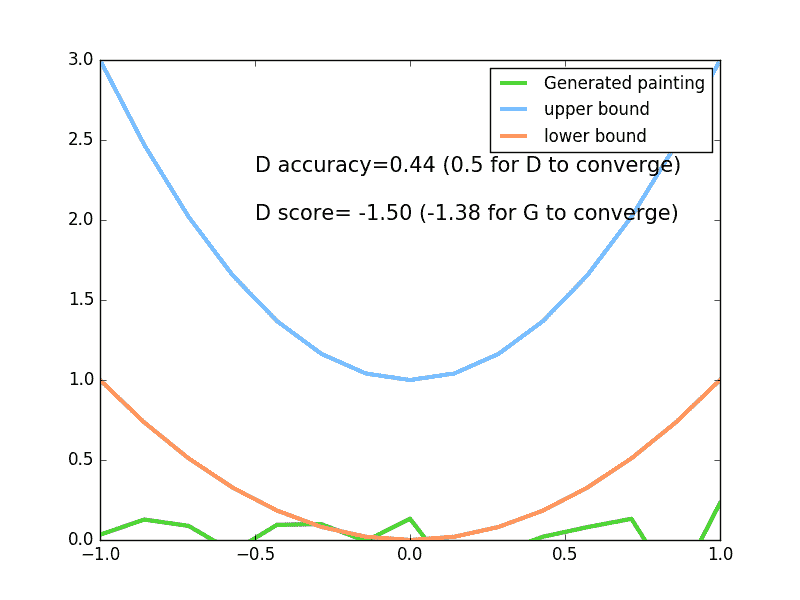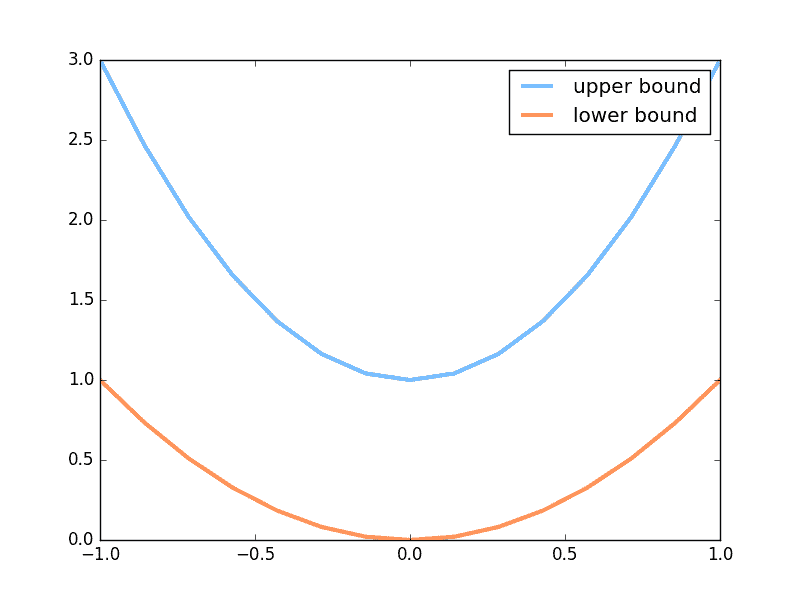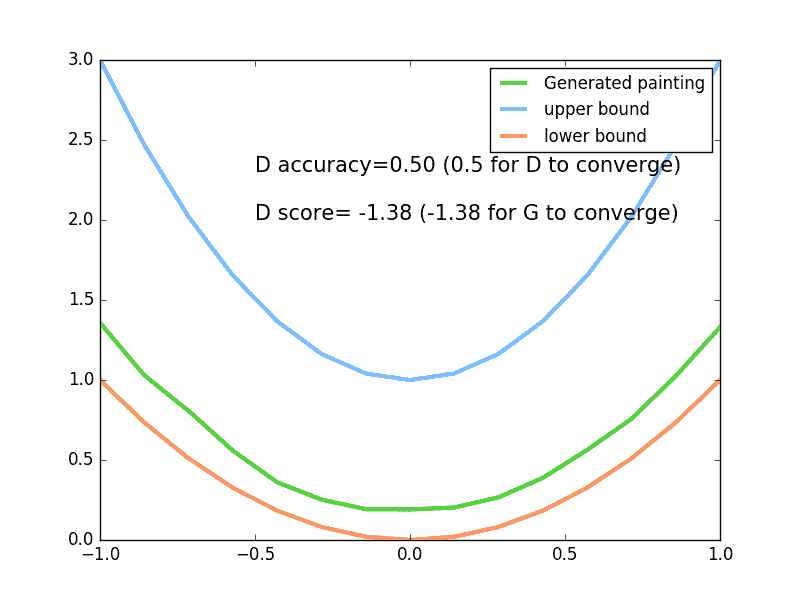# 4.6 – GAN (Generative Adversarial Nets 生成对抗网络)

GAN 是一个近几年比较流行的生成网络形式. 对比起传统的生成模型, 他减少了模型限制和生成器限制, 他具有有更好的生成能力. 人们常用假钞鉴定者和假钞制造者来打比喻, 但是我不喜欢这个比喻, 觉得没有真实反映出 GAN 里面的机理.## 超参数设置

import torch
import torch.nn as nn
import numpy as np
import matplotlib.pyplot as plt

torch.manual_seed(1)    # reproducible
np.random.seed(1)

# 超参数
BATCH_SIZE = 64
LR_G = 0.0001           # learning rate for generator
LR_D = 0.0001           # learning rate for discriminator
N_IDEAS = 5             # think of this as number of ideas for generating an art work (Generator)
ART_COMPONENTS = 15     # it could be total point G can draw in the canvas
PAINT_POINTS = np.vstack([np.linspace(-1, 1, ART_COMPONENTS) for _ in range(BATCH_SIZE)])


## 著名画家的画

def artist_works():     # painting from the famous artist (real target)
a = np.random.uniform(1, 2, size=BATCH_SIZE)[:, np.newaxis]
paintings = a * np.power(PAINT_POINTS, 2)   (a-1)
paintings = torch.from_numpy(paintings).float()
return Variable(paintings)## 神经网络

D 会接收一幅画作 (一元二次曲线), 输出这幅画作到底是不是著名画家的画(是著名画家的画的概率).

G = nn.Sequential(                      # Generator
nn.Linear(N_IDEAS, 128),            # random ideas (could from normal distribution)
nn.ReLU(),
nn.Linear(128, ART_COMPONENTS),     # making a painting from these random ideas
)

D = nn.Sequential(                      # Discriminator
nn.Linear(ART_COMPONENTS, 128),     # receive art work either from the famous artist or a newbie like G
nn.ReLU(),
nn.Linear(128, 1),
nn.Sigmoid(),                       # tell the probability that the art work is made by artist
)


## 训练

for step in range(10000):
artist_paintings = artist_works()           # real painting from artist
G_ideas = Variable(torch.randn(BATCH_SIZE, N_IDEAS))    # random ideas
G_paintings = G(G_ideas())                  # fake painting from G (random ideas)

prob_artist0 = D(artist_paintings)          # D try to increase this prob
prob_artist1 = D(G_paintings)               # D try to reduce this prob


    D_loss = - torch.mean(torch.log(prob_artist0)   torch.log(1\. - prob_artist1))
G_loss = torch.mean(torch.log(1\. - prob_artist1))


    opt_D.zero_grad()
D_loss.backward(retain_variables=True)      # retain_variables 这个参数是为了再次使用计算图纸
opt_D.step()